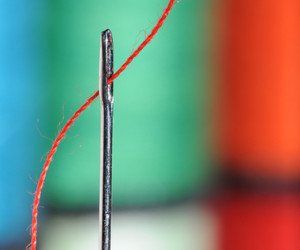# July 2013

## Calculating Side and Corner TrianglesSetting quilt blocks on point in a diagonal setting creates triangular spaces on the edges and in the corners that need to be filled in to make the quilt square. Use these simple calculations to determine what size triangles to cut for those spaces.

Calculating Side Triangles

Lay the quilt blocks out on the floor, or put them on a design wall, arranged in the desired diagonal setting.

Count how many side triangles are necessary. Do not include the corners.

The total number of triangles = (a).

Divide the total number of triangles by 4 = (b). Round up to the nearest whole number. This gives the number of squares of fabric you will need to cut.

Take the diagonal measurement of a block used in the quilt = (c). Add 2 inches to that measurement. The total = (d).

Cut the number of squares of fabric (b) to the measurement in (d). For example if (b) is 6 squares and your measurement (d) is 15 inches, you will cut 6 – 15 inch squares.

Cut each square into quarters diagonally.

Lay these triangles around the edges of the quilt.

Calculating Corner Triangles

Cut two squares of fabric the size of your blocks, plus 1 1/2 inches. For example, if the blocks in the quilt are 12 inches, cut two 13 1/2 inch blocks. Cut these in half diagonally

Place these triangles in the corners of the quilt.

Note: These triangles will be slightly larger than necessary, but the excess can be trimmed away when the borders or binding is added, ensuring that the edges of the quilt will be even and square.

Using some simple math calculations, you can easily determine the size of the triangles to cut for finishing your diagonal setting. Experiment with using a different color or print in the side and corner triangles to make the blocks appear as if they are floating on the background.

Until next time,

Joan

## Learn How to Hand Quilt